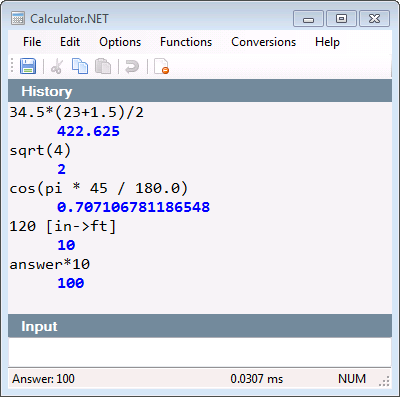{{ message }}
/ Calculator Public

Calculator.NET - Calculator that evaluates math expressions

# loresoft/Calculator

Switch branches/tags
Nothing to show

## Files

Failed to load latest commit information.
Type
Name
Commit time

# Calculator.NET

Calculator.NET - Calculator that evaluates math expressionsThe library supports math expressions, functions unit conversion and variables. Below are some examples of using the library directly.

``````MathEvaluator eval = new MathEvaluator();
//basic math
double result = eval.Evaluate("(2 + 1) * (1 + 2)");
//calling a function
result = eval.Evaluate("sqrt(4)");
//evaluate trigonometric
result = eval.Evaluate("cos(pi * 45 / 180.0)");
//convert inches to feet
result = eval.Evaluate("12 [in->ft]");
//use variable
result = eval.Evaluate("x * 10");
``````

Calculator that evaluates math expressions.### Calculator.NET Features

• Evaluate math expressions including grouping
• Support trigonometry and other function
• Common unit conversion of the following types
• Length
• Mass
• Speed
• Temperature
• Time
• Volume
• Variable support including last answer

Calculator.NET - Calculator that evaluates math expressions

1.2.0.42 Latest
Jun 9, 2015

## Packages 0

No packages published

•
•